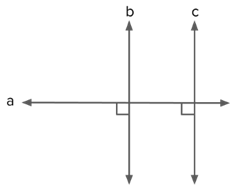QuestionAnswers

# If $a,b\,\,{\rm{and}}\,c$ are lines such that $a\, \bot b$ and $b||c$, then __________.VerifiedIf $a \bot b\,\,{\rm{and}}\,\,b||c \Rightarrow a \bot c$.